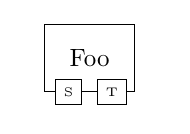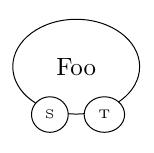# Subnodes on border of ellipsis

I want to place some subnodes on the border of a node. This is what I have:

\documentclass{article}

\usepackage{tikz}
\usetikzlibrary{positioning}

\begin{document}

\begin{tikzpicture}
\node[rectangle, draw, inner sep=10pt] (Foo) {Foo};
\node[rectangle, draw, fill=white, left=3pt of Foo.south] {\tiny S};
\node[rectangle, draw, fill=white, right=3pt of Foo.south] {\tiny T};
\end{tikzpicture}
\end{document}


Which nicely yields:But now I want to use ellipses as shapes:

\documentclass{article}

\usepackage{tikz}
\usetikzlibrary{positioning,shapes}

\begin{document}

\begin{tikzpicture}
\node[ellipse, draw, inner sep=10pt] (Foo) {Foo};
\node[ellipse, draw, fill=white, left=3pt of Foo.south] {\tiny S};
\node[ellipse, draw, fill=white, right=3pt of Foo.south] {\tiny T};
\end{tikzpicture}
\end{document}


And, unsurprisingly, the subnodes are not placed on the border anymore, but somewhat below (but they're still large enough to lap over the base node):How would I place those subnodes exactly on the border of the base node?

• this is not surprise ... your sub nodes position coordinate is wrong calculated. at ellipse they are not on the horizontal line through foo.fouth but higher, depend of ellipse curve. you need to determine coordinates of sub nodes accordingly. – Zarko Nov 17 '16 at 9:59
• Yes I know, the question is: how do I do that? – flyx Nov 17 '16 at 10:07
• one way is calculate intersection between ellipse any and path from ellipse center through ellipse border. – Zarko Nov 17 '16 at 10:12

\node[ellipse, draw, fill=white] at (Foo.-70) {\tiny T};

can be sufficient. If needed you can then use shift=(<x amount>,<y amount>) for fine tuning.